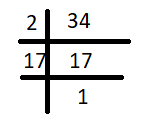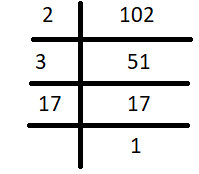Courses
Courses for Kids
Free study material
Offline Centres
MoreLast updated date: 28th Nov 2023
Total views: 290.4k
Views today: 3.90k

# Find the HCF of 34 and 102.Verified
290.4k+ views
Hint: Find the prime factorization of 34 and 102. Then choose all the factors which are common in both the prime factorization of 34 and the prime factorization of 102. Multiply these common factors to get the HCF of the two numbers.

We need to find the HCF of 34 and 102.
First let us see the definition of HCF.
The HCF or Highest Common Factor of two or more integers, which are not all zero, is the largest positive integer that divides each of the integers.
To find HCF of 34 and 102, we need to find their prime factorizations.
First, we will find the prime factorization of 34.So 34 can be written as: $34=2\times 17$
Similarly we now do the prime factorization of 102So 102 can be written as: $102=2\times 3\times 17$
Now, we need to find the factors which are common in both: the prime factorization of 34 and the prime factorization of 102.
We see that $2\times 17$ is common in both the prime factorizations.
So, the HCF is $2\times 17=34$.
Hence, the HCF of 34 and 102 is 34.

Note: HCF is the product of all the common factors of two or more numbers. We find this by doing prime factorization of each number and selecting the common factors.
If we have two prime numbers, then their prime factorization will have no common factor. In this case the HCF will be 1. So the HCF of two or more prime numbers is 1.